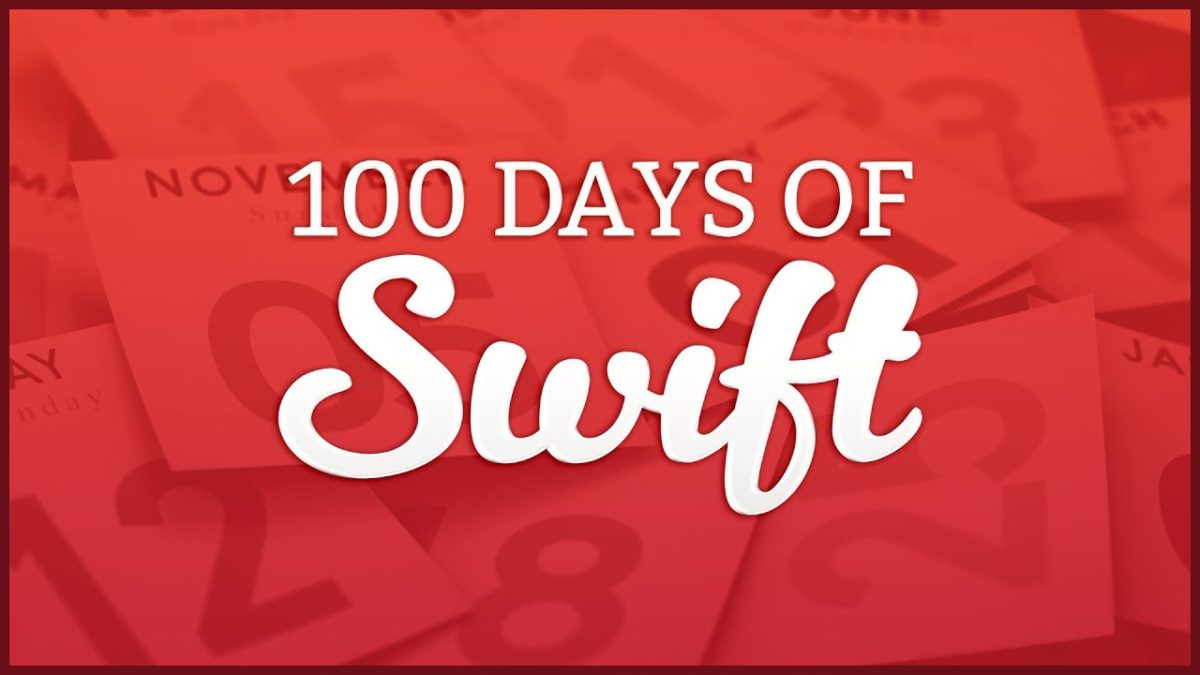# 100 Days of SwiftUI – Day 5 – FunctionsVariadic is a new term for me. The ability to pass many things into your function by using…

func square(numbers: Int…) {
for number in numbers {
print(“\(number) squared is \(number * number)”)
{
{

square(numbers: 1,2,3,4,5)

1 squared is 1
2 squared is 4
3 squared is 9
4 squared is 16
5 squared is 25

This is something I haven’t used before but I might in the future.